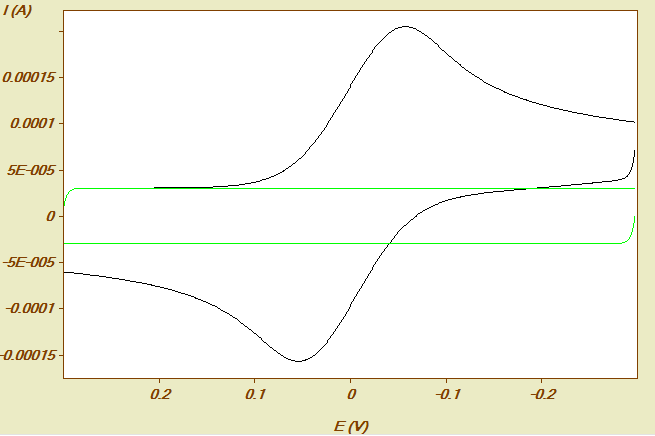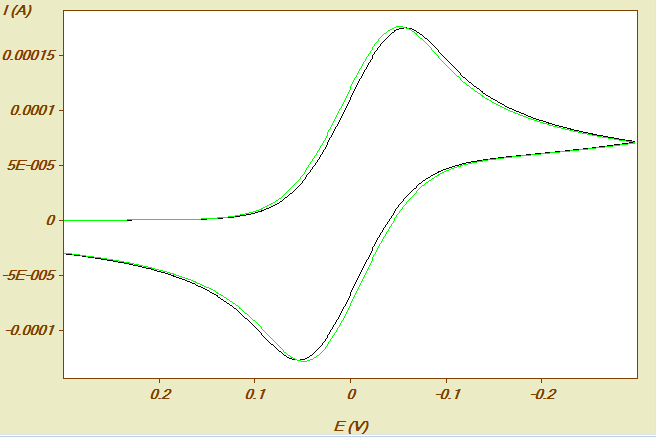﻿ Apply Background Correction in Simulations
DigiElch Help

Apply Background Correction in Simulations

 Apply Background Correction in SimulationsApply Background Correction in SimulationsIf this option is selected on the Property Page: Simulation Parameters the program performs a direct one-step simulation of a background-corrected experiment.
To provide an example, we consider a cyclic voltammetric experiment executed with no or incomplete IR-compensation. The experiment was conducted at a mercury drop electrode (referring to a drop weight of 4 mg) using a scan rate of 100 V/s. The double layer capacity of the electrode amounts to 300 nF and the uncompensated resistance to 100 Ohm. The concentration of the electrochemically active species is 0.001 M/l and the charge transfer is assumed to proceed in a fully reversible way. The black curve in the following picture shows the theoretical CV that would be measured for such a system.The green curve in the above picture refers to the charging current that would be theoretically obtained in the absence of the electrochemically active species. Background correction (subtracting the green curve from the black one) leads to the black CV shown in the following picture.Exactly the same curve would be directly simulated in one step when selecting "apply background correction" on the Simulation Parameters page.
Note that the effect of the charging current cannot by removed in the naive way by executing a simulation setting the double layer capacity of the electrode simply to zero. The resulting CV (shown by the green curve in the above picture) is not identical with the black one because the effect of the charging current on the overall IR-drop is not taken into account when proceeding in this way.
The direct one-step simulation of background-corrected curves becomes important when using (background-corrected) experimental curves with no or incomplete IR-compensation in a Data Fitting Project.